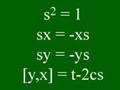18.735 | Fall 2009 | Graduate

# Double Affine Hecke Algebras in Representation Theory, Combinatorics, Geometry, and Mathematical Physics

## Four relations: s^2=1, sx=-xs, sy=ys, and [y,x] = t-2cs

Description:

The four relations that define the simplest Cherednik algebra. (Image by MIT OpenCourseWare.)

Alt text:
Four relations: s^2=1, sx=-xs, sy=ys, and [y,x] = t-2cs.
Caption:
The four relations that define the simplest Cherednik algebra. (Image by MIT OpenCourseWare.)